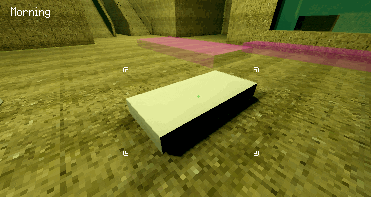Hi,

First question, hopefully this is clear, i want to do a item outline in the style of the original deus ex.What would be the correct way/ways to approach this ?

Godot version 3.4+ or 3+5
in Engine

+1 vote
• Create a NinePatchRect node. Set its anchors to Center in the menu at the top of the 2D editor viewport.
• Assign a nine-patch texture that gives it corners.
• In `_process()`, adjust the NinePatchRect's `rect_size` property depending on the distance between the camera. When the camera is further away from the object, you want the outline to be smaller to reflect the object's size on screen.
• In `_process`, use `Camera.unproject_position()` on the current camera to move the NinePatchRect according to the object's position. Take the NinePatchRect's `rect_size` into account so the outline is centered around the object. `get_viewport().get_camera()` may be helpful here (`get_viewport().get_camera_3d()` in 4.0).
by (12,908 points)
+1 vote

Late answer but I had the same problem and got it to work with something super accurate to the model.

First you want 8 corners of the target 3D object, you do this by finding the endpoints of the AABB by using a function like this on a target mesh instance. Might be less processing to grab the mesh of the area/body but this works fine:

``````func get_aabb_global_endpoints(mesh_instance: MeshInstance) -> Array:
if not is_instance_valid(mesh_instance):
return []

var mesh: Mesh = mesh_instance.mesh
if not mesh:
return []

var aabb: AABB = mesh.get_aabb()
var global_endpoints := []
for i in range(8):
var local_endpoint: Vector3 = aabb.get_endpoint(i)
var global_endpoint: Vector3 = mesh_instance.to_global(local_endpoint)
global_endpoints.push_back(global_endpoint)
return global_endpoints
``````This will return you an array of 8 Vector3 coordinates. Then you want to convert those into 2D coordinates using camera.unproject_position and put them in another size 8 array.

``````var cornerarray = get_aabb_global_endpoints(target)
var corner2D = []
for i in 8:
corner2D.push_front(camera.unproject_position(cornerarray[i]))
``````

(target in this snippet being the mesh instance)

This is the important part. We then want to figure out which of the 8 Vector2 coordinates that have the min/max of x/y coordinates . So this function will return 4 coordinates that fit that criteria:

``````func get_min_max_positions(cornerarray):
var min_x = cornerarray.x
var min_y = cornerarray.y
var max_x = cornerarray.x
var max_y = cornerarray.y
for i in 8:
if cornerarray[i].x < min_x:
min_x = cornerarray[i].x
if cornerarray[i].y < min_y:
min_y = cornerarray[i].y
if cornerarray[i].x > max_x:
max_x = cornerarray[i].x
if cornerarray[i].y > max_y:
max_y = cornerarray[i].y

return [Vector2(min_x,min_y),Vector2(min_x,max_y),Vector2(max_x,min_y),Vector2(max_x,max_y)]
``````

This will give you 4 sets of 2D coordinates of where the corners should be, so it could be corners of a rect or in my case 4 L shaped corner sprites, whatever works.

``````var minMax = get_min_max_positions(corner2D)
for i in 4:
\$cornerIcons.get_child(i).position = minMax[i]
``````

so something like this will give me this result:by (21 points)